# “Greater Than or Equal to” (>=) In ExcelArticle byExcelMojo Team## What Is The “Greater Than Or Equal To” (>=) In Excel?

The “greater than or equal to” (>=) in Excel is a logical operator used for comparing two values of the same data type. It returns TRUE if the first data is greater than or equal to the second value. Otherwise, the outcome is FALSE. Users can use this operator to compare numeric values, dates, time, and text data.

For example, the below table shows two sets of data.

Suppose we need to compare the data points of the two data sets in rows 2, 3, and 4 and determine the value “greater than or equal to” in Excel. Then, using greater than or equal to in Excel logical operator, we can get the required output.

Also, we will see how we can use the logical operator “greater than or equal to” (>=) in Excel in an IF() in row 6.

Here, a later date or time is greater than an earlier date or time, respectively. On the other hand, a letter that appears later in the alphabet series is greater than the one appearing in the beginning.

Likewise, we can insert “greater than or equal to” (>=) in Excel functions such as COUNTIF and SUMIF. We can use it in their test conditions when they check if one value is greater than the other or if both values are equal. In that case, they return TRUE.

###### Key Takeaways
• The logical operator, “greater than or equal to” (>=) in Excel, compares two values and returns TRUE if the first data is equal to or more than the second data. Otherwise, the operator returns FALSE.
• We can directly use the “greater than or equal to” logical operator in a formula cell.
• The two values we compare using the “greater than or equal to” logical operator should be of the same data type.
• We can apply the “greater than or equal to” logical operator in the test conditions in functions such as IF, COUNTIF, and SUMIF.

### How To Use “Greater Than” (>) And “Greater Than Or Equal To” (>=)?

The steps to use the logical operator, “greater than or equal to” (>=) in Excel, are:

1. Ensure we have all the required data points to compare or use to test conditions in functions such as IF and COUNTIF in our spreadsheet.
2. Choose the target cell and type the ‘=’ sign followed by the first value or cell reference to the first value. Then, enter the “greater than or equal to” (>=) logical operator and the second value or a cell reference to it. And suppose we need to use the operator in a function to determine the value “greater than or equal to” (>=) in Excel compared to another value, then enter the function in the target cell with the operator inserted in the test condition.
3. Press Enter to view the value of the operator or function.

Please Note: The above steps also apply to the “greater than” (>) logical operator. But the only difference is that the symbol we need to insert should be ‘>’ instead of the symbol for “greater than or equal to” in excel

Here is an illustration to explain the steps.

Consider the below table. It shows students’ overall scores, with a score greater or equal to 50 as the pass criterion. So, let us see how to use the two logical operators for the given condition.

The first two steps show how to use the “greater than” (>) logical operator.

Step 1: Choose cell B2 and type the formula containing the “greater than” logical operator, i.e., =A2>50

Press Enter.

Step 2: Drag the Autofill handle downwards to copy the formula in the range B2:B10.

The following two steps show how to use the logical operator “greater than or equal to” (>=) in Excel.

Step 3: Choose cell C2 and type the “greater than or equal to” (>=) in Excel formula, i.e., =A2>=50.

Press Enter.

Step 4: Drag the fill handle downwards to copy the above “greater than or equal to” (>=) in Excel formula in cell range C2:C10.

While the two logical operators return the same results in most cases, the output varies for a score of 50 (Refer to rows 7 and 9 in the above image).

The reason is that the “greater than” operator returns TRUE only if the score exceeds 50. But the operator “greater than or equal to” (>=) in Excel checks if the score is greater or equal to 50 to return TRUE.

### Examples

Here are some scenarios where we can insert “greater than or equal to” (>=) in Excel functions and use it as a comparison operator.

#### Example #1- “Greater Than Or Equal To” With The IF Function

Assume we need to update the bonus eligibility status in the below table. The criteria are that if a branch office sells more than or equal to 1000000 units, it is eligible for a bonus.

The steps to use the IF() with the logical operator, “greater than or equal to” in excel formula are:

Step 1: Choose cell C2 and type the IF() formula,

=IF(B2>=1000000,”Eligible”,”Not Eligible”).

Press Enter.

Step 2: Drag the fill handle downwards to copy the IF Excel function containing the logical operator, “greater than or equal to” (>=) in Excel, in the cell range C3:C6.

Since the IF() condition contains the symbol for “greater than or equal to” in excel, Branch Office 4 is eligible for a bonus, as it sold precisely 1000000 units.

#### Example #2-“Greater Than Or Equal to” With The COUNTIF Function

Consider the below table showing a list of items, their order dates, and the quantities ordered.

Suppose we need to determine the number of items with orders for 200 or more units and the number of items ordered in March and April. Then, we can apply the COUNTIF() with the test condition using the logical operator “greater than or equal to” (>=) in Excel.

Let us display the required results in cells F1 and F2.

Step 1: Choose cell F1 and type the COUNTIF() formula

=COUNTIF(B2:B16,”>=200″)

Press Enter.

The COUNTIF() with the logical operator, “greater than or equal to” in Excel formula counts those cells with a value of 200 or more in the cell range B2:B16 and returns the total count.

Step 2: Choose cell F2 and type the following COUNTIF() formula:

=COUNTIF(C2:C16,”>=1/3/2021″)-COUNTIF(C2:C16,”>=1/5/2021″)

Press Enter.

The above formula uses the COUNTIF() twice, with the first function testing the condition with the “greater than or equal to” logical operator. The two COUNTIF functions count all the order dates on and after 3/1/2021 and 5/1/2021, respectively. So, when we find the difference between the two function outputs, we will get the required count.

Thus, the “greater than or equal to” logical operator avoids the possible ambiguity with the dates, helping us decide whether to include or exclude a specific date during calculations.

#### Example #3- “Greater Than Or Equal to” With The SUMIF Function

The below table shows sales representatives and their achieved sales targets.

Suppose we need to determine the total of all the sales amount higher than \$15000 and display the result in cell E2. Then, we can apply the SUMIF() with the test condition based on the logical operator, “greater than or equal to” (>=) in Excel.

Step 1: Choose cell E2 and type the SUMIF() excel formula,

=SUMIF(B2:B11,”>=15000″)

Press Enter.

Here, the test condition in the SUMIF() checks for those sales values that are equal to or more than \$15000, as the logical operator is “greater than or equal to” (>=) in Excel.

And then, the function adds those sales amounts which satisfy the criterion.

Can you use greater than or equal to in Excel?

We can apply greater than or equal to in Excel as a logical operator to compare two values of the same data type. The symbol is >=.

Further, we can use it in functions such as IF, COUNTIF, and SUMIF to test the conditions that decide their outcomes.

Where is greater than or equal to in Excel?

The greater than or equal to in Excel is not available as an inbuilt function. Instead, it is a logical operator that we need to enter in the target cell manually.

However, it is available as an option when applying conditional formatting. For example, we can navigate Home > Conditional Formatting > Highlight Cells Values > More Rules to open the New Formatting Rule window.

Here we can choose the logical operator to compare a cell data with another value to decide whether to format the specific cell.

How to type greater than or equal to in Excel?

We can type greater than or equal to in Excel by entering the greater than symbol, ‘>’, and then the equal to sign, ‘=’.

For example, if we use the greater than or equal to in a formula within a cell to compare two values in Excel, say 10 and 5, the expression in the target cell will be:

=10>=5

When we press Enter, we get the output as TRUE since the values satisfy the condition.

How do you use greater than or equal to in Excel to conditional formatting?

We can use greater than or equal to in Excel to conditional formatting by following the below steps.

Suppose the table below contains the source data, and we need to highlight the cells in column B with fruit units sold values greater or equal to 1000. Using greater than or equal to in excel, we can find the value.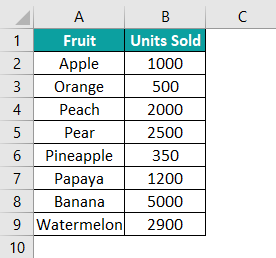Step 1: Choose the cell range B2:B9 and select Home > Conditional Formatting > Highlight Cells Values > More Rules.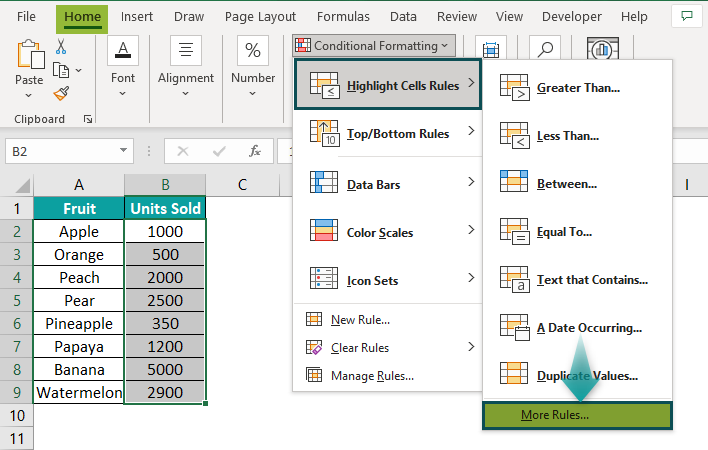Step 2: The New Formatting Rule window pops up. Here, we can choose the required logical operator from the drop-down list as depicted in the image below.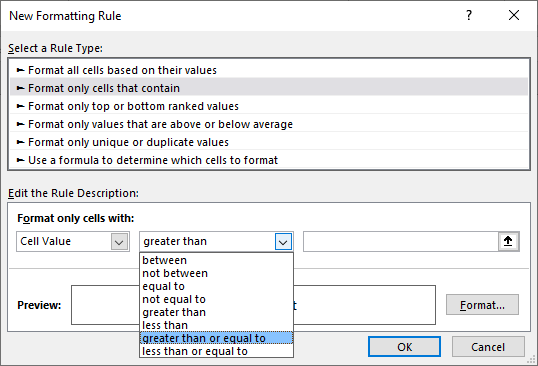Step 3: Enter the value we need to compare with the data in cell range B2:B9, as depicted in the image below.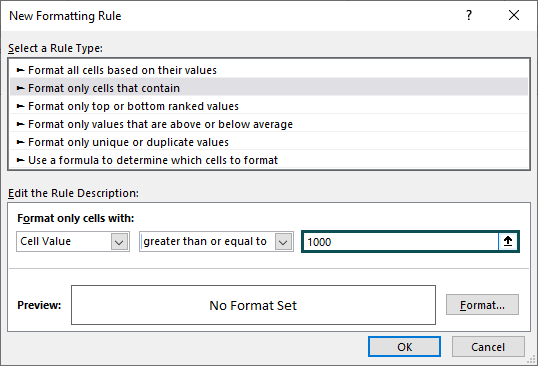Step 4: Next, click on Format. The Format Cells window appears.

Here, we need to click on the Fill tab.

Choose the shade from the color palette we want to use to highlight the cells that satisfy the test condition, and click OK.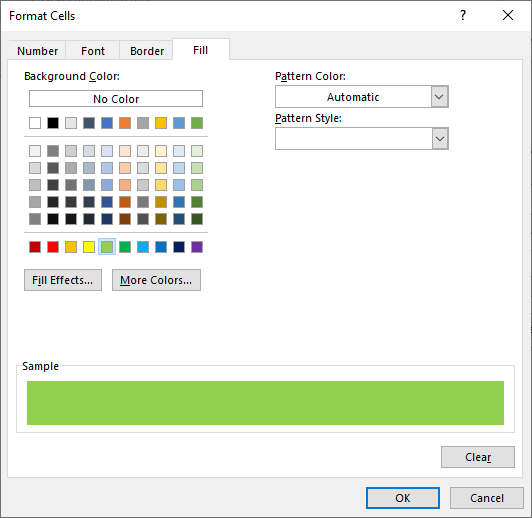Step 5: Check the format preview in the New Formatting Rule window and click OK to close it.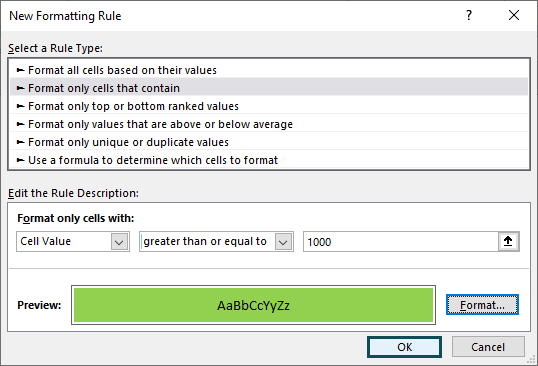Once we click OK, the outcome will be returned as shown in the image below: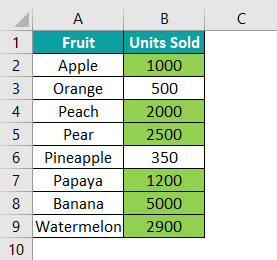Those cells in column B with values greater than or equal to 1000 get highlighted in the chosen shade.

Similarly, we can make use of the logical operator to obtain results in excel.